## How to Calculate and Solve for Equilibrium Constant for Free Energy | Corrosion

The equilibrium constant for free energy is illustrated by the image below.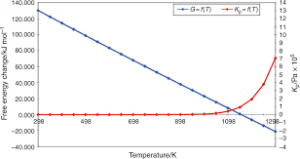To compute for equilibrium constant for free energy, three essential parameters are needed and these parameters are Change in Free Energy (ΔG°), Gas Constant (R) and Temperature (T).

K = exp(ΔG°/RT)

Where:

ΔG° = Change in Free Energy
R = Gas Constant
T = Temperature

Let’s solve an example;
Find the equilibrium constant for free energy when the change in free energy is 14, the gas constant is 10 and the temperature is 2.

This implies that;

ΔG° = Change in Free Energy = 14
R = Gas Constant = 10
T = Temperature = 2

K = exp(ΔG°/RT)
K = exp(14/(10)(2))
K = exp(14/20)
K = exp(0.7)
K = 2.0137

## How to Calculate and Solve for Rate Expansion of Metal | Corrosion

The rate expansion of metal is illustrated by the image below.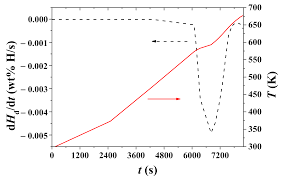To compute for rate expansion of metal, two essential parameters are needed and these parameters are Time Independent Constant (K) and Time of Expansion (t).

The formula for calculating the rate expansion of metal:

W = Kt

Where:

W = Rate Expansion of Metal
K = Time Independent Constant
t = Time of Expansion

Let’s solve an example;
Find the rate expansion of metal when the time independent constant is 48 and the time of expansion is 12.

This implies that;

K = Time Independent Constant = 48
t = Time of Expansion = 12

W = Kt
W = (48)(12)
W = 576

Therefore, the rate expansion of metal is 576.

Calculating the Time Independent Constant when the Rate Expansion of Metal and the Time of Expansion is Given.

K = W / t

Where:

K = Time Independent Constant
W = Rate Expansion of Metal
t = Time of Expansion

Let’s solve an example;
Find the time independent constant when the rate expansion of metal is 36 and the time of expansion is 9.

This implies that;

W = Rate Expansion of Metal = 36
t = Time of Expansion = 9

K = W / t
K = 36 / 9
K = 4

Therefore, the time independent constant is 4.

## How to Calculate and Solve for Pilling Bedworth Ratio | Corrosion

The pilling bedworth ratio is illustrated by the image below.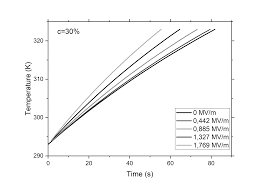To compute for pilling bedworth ratio, four essential parameters are needed and these parameters are Weight of the Oxide (Ao), Weight of the Metal (Am), Density of the Oxide (ρo) and Density of the Metal (ρm).

The formula for calculating pilling bedworth ratio:

PBR = Aoρm/Amρo

Where:

PBR = Pilling-Bedworth Ratio
Ao = Weight of the Oxide
Am = Weight of the Metal
ρo = Density of the Oxide
ρm = Density of the Metal

Let’s solve an example;
Find the pilling bedworth ratio when the weight of the oxide is 21, the weight of the metal is 7, the density of the oxide is 14 and the density of the metal is 2.

This implies that;

Ao = Weight of the Oxide = 22
Am = Weight of the Metal = 7
ρo = Density of the Oxide = 14
ρm = Density of the Metal = 2

PBR = Aoρm/Amρo
PBR = (21)(2)/(7)(14)
PBR = (42)/(98)
PBR = 0.428

Therefore, the pilling bedworth ratio is 0.428.

Calculating the Weight of the Oxide when the Pilling Bedworth Ratio, the Weight of the Metal, the Density of the Oxide and the Density of the Metal is Given.

Ao = PBR x Amρo / ρm

Where:

Ao = Weight of the Oxide
PBR = Pilling-Bedworth Ratio
Am = Weight of the Metal
ρo = Density of the Oxide
ρm = Density of the Metal

Let’s solve an example;
Given that the pilling bedworth ratio is 10, the weight of the metal is 4, the density of the oxide is 2 and the density of the metal is 8. Find the weight of the oxide?

This implies that;

PBR = Pilling-Bedworth Ratio = 10
Am = Weight of the Metal = 4
ρo = Density of the Oxide = 2
ρm = Density of the Metal = 8

Ao = PBR x Amρo / ρm
Ao = 10 x (4)(2) / 8
Ao = 10 x 8 / 8
Ao = 80 / 8
Ao = 10

Therefore, the weight of the oxide is 10.

## How to Calculate and Solve for Exchange Current Density | Corrosion

The exchange current density is illustrated by the image below.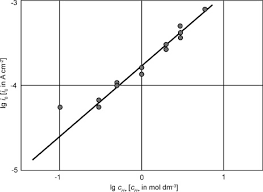To compute for exchange current density, three essential parameters are needed and these parameters are Current Density (io), Number of Electrons (n) and Faraday’s Constant (F).

The formula for calculating exchange current density:

γredoxid) = io/nF

Where:

γredoxid) = Exhange Current Density
io = Current Density
n = Number of Electrons

Given an example;
Find the exchange current density when the current density is 8, the number of electrons is 4 and the faraday’s constant is 2.

This implies that;

io = Current Density = 8
n = Number of Electrons = 4
F = Faraday’s Constant = 2

γredoxid) = io/nF
γredoxid) = 8/(4)(2)
γredoxid) = 8/(8)
γredoxid) = 1

Therefore, the exchange current density is 1.

## How to Calculate and Solve for Activation Polarization | Corrosion

The activation polarization is illustrated by the image below.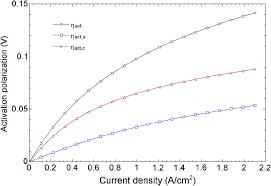To compute for activation polarization, three essential parameters are needed and these parameters are Constant for the Half Cell (β), Constant for the Half Cell (io) and Current per Unit Density (i).

The formula for calculating activation polarization:

ηa = ±β log(i/io)

Where:

ηa = Activation Polarization
β = Constant for the Half Cell
io = Constant for the Half Cell
i = Current per Unit Density

Let’s solve an example;
Find the activation polarization when the constant for the half cell is 10, the constant for the half cell is 5 and the current per unit density is 20.

This implies that;

β = Constant for the Half Cell = 10
io = Constant for the Half Cell = 5
i = Current per Unit Density = 20

ηa = ±β log(i/io)
ηa = ±(10) log(20/5)
ηa = ±(10) log(4)
ηa = ±(10) (0.6020)
ηa = ±6.020

Therefore, the activation polarization is ±6.020.

## How to Calculate and Solve for Corrosion Rate | Corrosion

The corrosion rate is illustrated by the image below.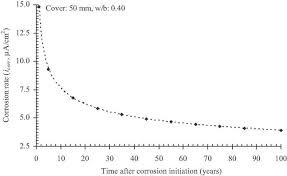To compute for corrosion rate, three essential parameters are needed and these parameters are Current per Unit Time (i), Number of Electrons (n) and Faraday’s Constant (F).

The formula for calculating corrosion rate:

r = i/nF

Where:

r = Corrosion Rate
i = Current per Unit Time (Current Density)
n = Number of Electrons

Given an example;
Find the corrosion rate when the current per unit time is 15, the number of electrons is 5 and the faraday’s constant is 3.

This implies that;

i = Current per Unit Time (Current Density) = 15
n = Number of Electrons = 5
F = Faraday’s Constant = 3

r = i/nF
r = 15/(5)(3)
r = 15/15
r = 1

Therefore, the corrosion rate is 1.

Calculating the Current per Unit Time when the Corrosion Rate, the Number of Electrons and the Faraday’s Constant is Given.

i = r (nF)

Where:

i = Current per Unit Time (Current Density)
r = Corrosion Rate
n = Number of Electrons

Let’s solve an example;
Find the current per unit time when the corrosion rate is 10, the number of electrons is 8 and the faraday’s constant is 3.

This implies that;

r = Corrosion Rate = 10
n = Number of Electrons = 8
F = Faraday’s Constant = 3

i = r (nF)
i = 10 (8)(3)
i = 10 (24)
i = 240

Therefore, the current per unit time is 240.

## How to Calculate and Solve for Corrosion Penetration Rate | Corrosion

The corrosion penetration rate is illustrated by the image below.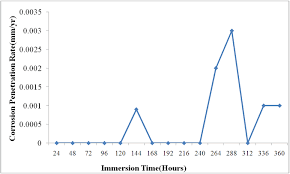To compute for corrosion penetration rate, five essential parameters are needed and these parameters are Constant (k), Weight Loss after Corrosion (W), Density of Corroding Media (ρ), Cross-sectional Area (A) and Time (t).

The formula for calculating corrosion penetration rate:

CPR = kW/ρAt

Where:

CPR = Corrosion Penetration Rate
k = Constant
W = Weight Loss after Corrosion
ρ = Density of Corroding Media
A = Cross-sectional Area
t = Time

Let’s solve an example;
Find the corrosion penetration rate when the constant is 2, the weight loss after corrosion is 4, the density of corroding media is 6, the cross-sectional area is 12 and time is 7.

This implies that;

k = Constant = 2
W = Weight Loss after Corrosion = 4
ρ = Density of Corroding Media = 6
A = Cross-sectional Area = 12
t = Time = 7

CPR = kW/ρAt
CPR = (2)(4)/(6)(12)(7)
CPR = 8/504
CPR = 0.0158

Therefore, the corrosion penetration rate is 0.0158.

## How to Calculate and Solve for Nernst Equation | Corrosion

The nernst equation is illustrated by the image below.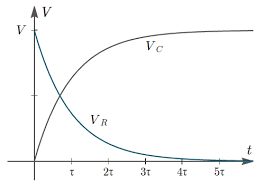To compute for nernst equation, seven essential parameters are needed and these parameters are Electrochemical Cell Potential (ΔV°), Gas Constant (R), Temperature (T), Number of Electrons (n), Faraday’s Constant (F), Molar Concentration [M1n+] and Molar Concentration [M2n+].

The formula for caluclating nernst equation:

ΔV = ΔV° – RT/nF In(M1n+/M2n+)

Where:

ΔV = Nernst Equation | Potential
ΔV° = Electrochemical Cell Potential
R = Gas Constant
T = Temperature
n = Number of Electrons
M1n+ = Molar Concentration
M2n+ = Molar Concentration

Let’s solve an example;
Find the nernst equation when the electrochemical cell potential is 8, the gas constant is 2, the temperature is 5, the number of electrons is 3, the faraday’s constant is 9, the molar concentration is 10 and the molar concentration is 4.

This implies that;

ΔV° = Electrochemical Cell Potential = 8
R = Gas Constant = 2
T = Temperature = 5
n = Number of Electrons = 3
F = Faraday’s Constant = 9
M1n+ = Molar Concentration = 10
M2n+ = Molar Concentration = 4

ΔV = ΔV° – RT/nF In(M1n+/M2n+)
ΔV = 8 – ((2)(5)/(3)(9)) In(10/4)
ΔV = 8 – (10/27) In (2.5)
ΔV = 8 – 0.37 (0.91)
ΔV = 8 – (0.339)
ΔV = 7.66

Therefore, the nernest equation is 7.66 V.

## How to Calculate and Solve for Electrochemical Cell Potential | Corrosion

The electrochemical cell potential is illustrated by the image below.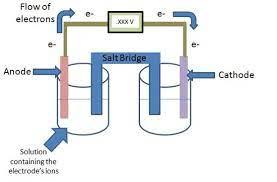To compute for electrochemical cell potential, two essential parameters are needed and these parameters are Positive Cell Potential (V2°) and Negative Cell Potential (V1°).

The formula for calculating electrochemical cell potential:

ΔV° = V2° – V1°

Where:

ΔV° = Electrochemical Cell Potential
V2° = Positive Cell Potential
V1° = Negative Cell Potential

Given an example;
Find the electrochemical cell potential when the positive cell potential is 14 and the negative cell potential is 7.

This implies that;

V2° = Positive Cell Potential = 14
V1° = Negative Cell Potential = 7

ΔV° = V2° – V1°
ΔV° = 14 – 7
ΔV° = 7

Therefore, the elecreochemical cell potential is 7 V.

Calculating the Positive Cell Potential when the Electrochemical Cell Potential and the Negative Cell Potential is Given.

V2° = ΔV° + V1°

Where:

V2° = Positive Cell Potential
ΔV° = Electrochemical Cell Potential
V1° = Negative Cell Potential

Let’s solve an example;
Find the positive cell potential when the electrochemical cell potential is 20 and the negative cell potential is 14.

This implies that;

ΔV° = Electrochemical Cell Potential = 20
V1° = Negative Cell Potential = 14

V2° = ΔV° + V1°
V2° = 20 + 14
V2° = 34

Therefore, the positive cell potential is 34 V.

## How to Calculate and Solve for Current Density | Corrosion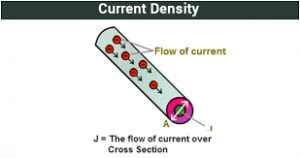The image above represents current density.

To compute for current density, three essential parameters are needed and these parameters are Number of Electron Charge Present (z), Force (F) and Rate of Heterogeneous Reaction (V).

The formula for calculating current density:

j = zFV

Where:

j = Current Density
z = Number of Electron Charge Present
F = Force
V = Rate of Heterogeneous Reaction

Let’s solve an example;
Find the current density when the number of electron charge present is 6, the force is 4 and the rate of heterogeneous reaction is 8.

This implies that;

z = Number of Electron Charge Present = 6
F = Force = 4
V = Rate of Heterogeneous Reaction = 8

j = zFV
j = (6) (4) (8)
j = 192

Therefore, the current density is 192 A/m².

Calculating the Number of Electron Charge Present when the Current Density, the Force and the Rate of Heterogeneous Reaction is Given.

z = j / FV

Where;

z = Number of Electron Charge Present
j = Current Density
F = Force
V = Rate of Heterogeneous Reaction

Let’s solve an example;
Find the number of electron charge present when the current density is 40, the force is 2 and the rate of heterogeneous reaction is 4.

This implies that;

j = Current Density = 40
F = Force = 2
V = Rate of Heterogeneous Reaction = 4

z = j / FV
z = 40 / 2 x 4
z = 40 / 8
z = 5

Therefore, the number of electron charge present is 5.